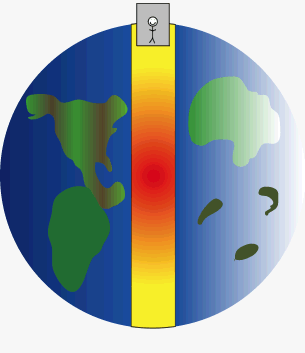Home » Ask Physics » Gravity

Gravity

We all know there is no such thing as zero gravity even at the very end of universe there is micro gravity according to formula for gravity…!
Means we are away from certain object so the gravity exist in vacuum so now my question is:-
Q- We all know that there is zero Gravity in center of earth core so if we place an object in center of earth the would Gravity formula apply on that object or that object is in complete Zero gravity…????

If we simply keep a body at the centre, it will remain there as it is attracted equally in all directions.A man in an elevator free falls through a planet to simulate how a gravity train could work. At both ends of the planet the train comes to a complete stop, but free falls through the middle (or wherever the tunnel is constructed) of the planet. (Photo credit: Wikipedia)

GRAVITYVisitors So Far @ AskPhysics

• 2,159,155 hits

Subscribe to Blog via EmailAsk Physics